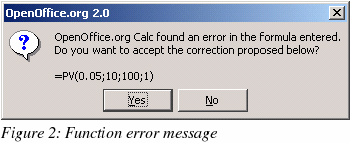On-line GuidesAll GuideseBook StoreiOS / AndroidLinux for BeginnersOffice ProductivityLinux InstallationLinux SecurityLinux UtilitiesLinux VirtualizationLinux KernelSystem/Network AdminProgrammingScripting LanguagesDevelopment ToolsWeb DevelopmentGUI Toolkits/DesktopDatabasesMail SystemsopenSolarisEclipse DocumentationTechotopia.comVirtuatopia.comHow To GuidesVirtualizationGeneral System AdminLinux SecurityLinux FilesystemsWeb ServersGraphics & DesktopPC HardwareWindowsProblem SolutionsPrivacy PolicyMicrosoft Office to OpenOffice Migration Guide
Previous Page Home Next Page

# Functions

CautionAn important difference between Excel and Calc regarding functions is that the values supplied to a function (which are called arguments in Excel and parameters in Calc) have different separators. Calc always uses semicolons to separate parameters in a function. Excel uses either commas or semicolons, depending on the system (for example commas on English systems, semicolons on German systems). Calc will generate a “#NAME?” error if you use a comma in place of a semi-colon.

Optional parameters in functions

Some formulas have more than one optional parameter at the end of the parameter list. In Excel it is possible to leave an optional parameter blank and specify a later parameter, but this is not possible in Calc. When using Calc functions, parameters marked as "optional" can be left out (or left empty) only when no parameter follows.

For example, to calculate an annuity by using the Present value function, PV(Rate; NPER; PMT; FV; Type), without the Future Value variable (FV):

• In Excel the formula =PV(0.05,10,100,,1) returns -\$810.78

• in Calc =PV(0.05;10;100;;1) returns Err:511 (Variable missing).

When you enter the PV function in Calc with the FV variable empty, a message box is displayed offering to change the incorrect input to “=PV(0.05;10;100;1):This, too, is incorrect as it will produce a value of -772.79.

The work-around is to put values in for the missing parameters. For the above example, putting a zero in for the blank parameter returns the correct result for the annuity, -\$810.78.

Date values in financial functions

In Excel some of the financial functions accept string parameters for dates; in Calc financial functions require a serial number, although you can simply type in the dates in date format without quotes.

Whenever an Excel Analysis ToolPak function has the same name as a Calc function, there is an equivalent in the Calc Analysis AddIn function but with a trailing _ADD. This ensures compatibility with Excel but without changing the behavior of Calcs built-in functions.

For example, the functions EFFECT and CONVERT provided by the Excel Analysis ToolPak are named EFFECT_ADD and CONVERT_ADD in Calc and return the same results.

The functions whose names end with _ADD return the same results as the corresponding Microsoft Excel functions. Use the functions without _ADD to get results based on international standards. For example, the WEEKNUM function calculates the week number of a given date based on international standard ISO 6801, while WEEKNUM_ADD returns the same week number as Microsoft Excel.

Differences in function results

Calc and Excel handle the missing Reference parameter with the Cell function, CELL(Info_type; Reference), differently. If Reference is missing, Calc uses the position of the cell in which this formula is located. Excel uses the reference of the cell in which the cursor is positioned.

Functions not implemented or imported

Excel functions which are not imported or implemented in Calc include INFO and GETPIVOTDATA. Excel has no equivalent for Calc’s ARABIC function which calculates the value of a Roman number.

There are issues when importing both the Ceiling function, CEILING (Number; Significance; Mode), and the Floor function, FLOOR(Number; Significance; Mode). If both parameters Number and Significance are negative and the Mode value is equal to zero or is not given, the results in OpenOffice.org and Excel will differ after the import has been completed. If you are exporting a Calc spreadsheet to Excel, use Mode=1 to see the same results in Excel as in Calc.

Microsoft Office to OpenOffice Migration Guide
Previous Page Home Next PagePublished under the terms of the GNU General Public License Design by Interspire